Скачать презентацию One can no more ban derivatives than the

cb10009cdbcd7a0f2b865859c8300bc4.ppt

• Количество слайдов: 21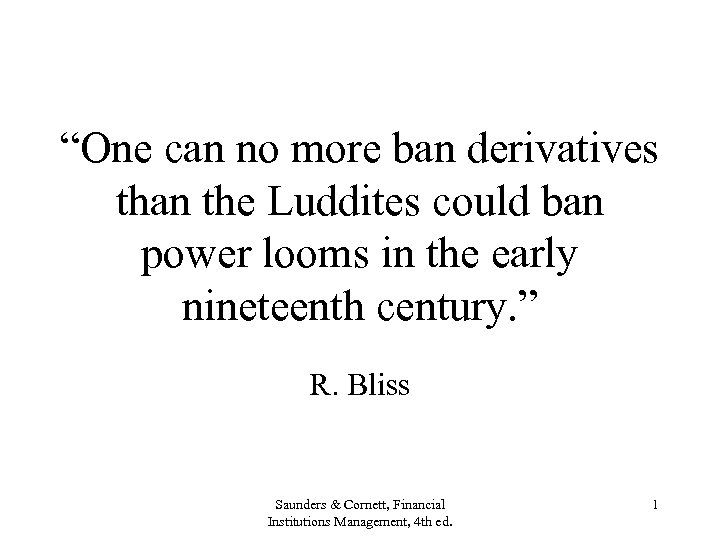“One can no more ban derivatives than the Luddites could ban power looms in the early nineteenth century. ” R. Bliss Saunders & Cornett, Financial Institutions Management, 4 th ed. 1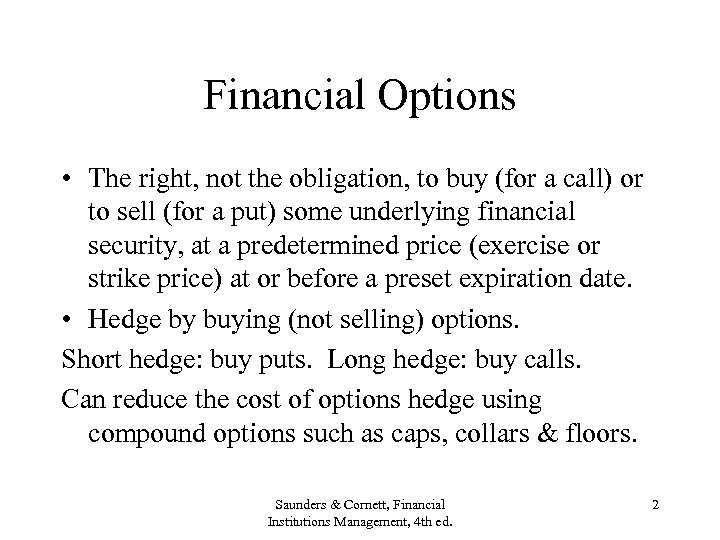Financial Options • The right, not the obligation, to buy (for a call) or to sell (for a put) some underlying financial security, at a predetermined price (exercise or strike price) at or before a preset expiration date. • Hedge by buying (not selling) options. Short hedge: buy puts. Long hedge: buy calls. Can reduce the cost of options hedge using compound options such as caps, collars & floors. Saunders & Cornett, Financial Institutions Management, 4 th ed. 2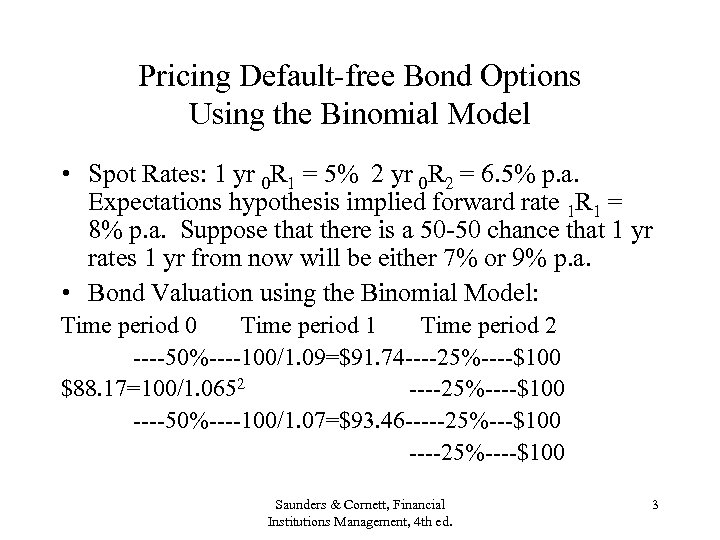Pricing Default-free Bond Options Using the Binomial Model • Spot Rates: 1 yr 0 R 1 = 5% 2 yr 0 R 2 = 6. 5% p. a. Expectations hypothesis implied forward rate 1 R 1 = 8% p. a. Suppose that there is a 50 -50 chance that 1 yr rates 1 yr from now will be either 7% or 9% p. a. • Bond Valuation using the Binomial Model: Time period 0 Time period 1 Time period 2 ----50%----100/1. 09=\$91. 74 ----25%----\$100 \$88. 17=100/1. 0652 ----25%----\$100 ----50%----100/1. 07=\$93. 46 -----25%---\$100 ----25%----\$100 Saunders & Cornett, Financial Institutions Management, 4 th ed. 3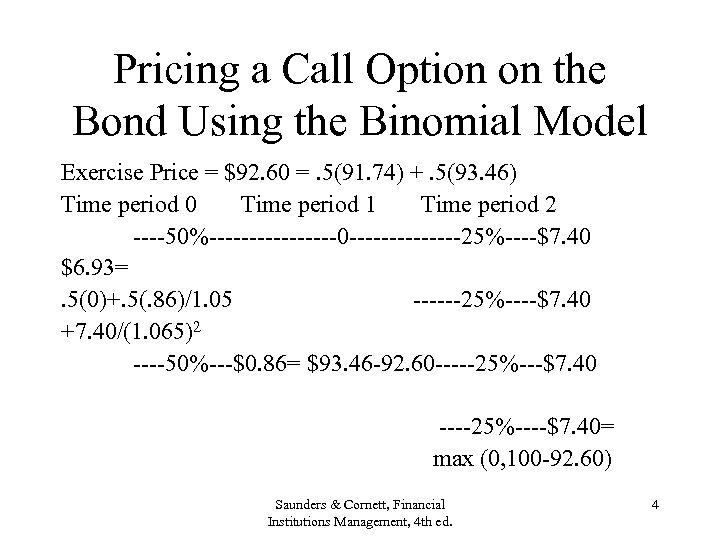Pricing a Call Option on the Bond Using the Binomial Model Exercise Price = \$92. 60 =. 5(91. 74) +. 5(93. 46) Time period 0 Time period 1 Time period 2 ----50%--------0 -------25%----\$7. 40 \$6. 93=. 5(0)+. 5(. 86)/1. 05 ------25%----\$7. 40 +7. 40/(1. 065)2 ----50%---\$0. 86= \$93. 46 -92. 60 -----25%---\$7. 40 ----25%----\$7. 40= max (0, 100 -92. 60) Saunders & Cornett, Financial Institutions Management, 4 th ed. 4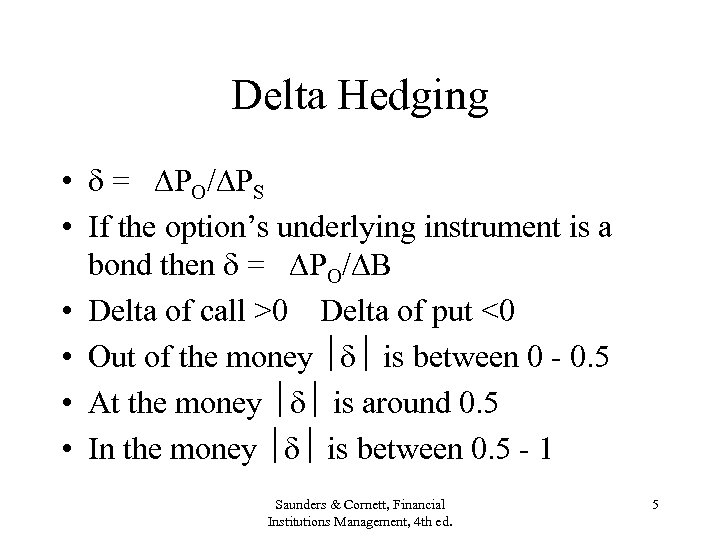Delta Hedging • = PO/ PS • If the option’s underlying instrument is a bond then = PO/ B • Delta of call >0 Delta of put <0 • Out of the money is between 0 - 0. 5 • At the money is around 0. 5 • In the money is between 0. 5 - 1 Saunders & Cornett, Financial Institutions Management, 4 th ed. 5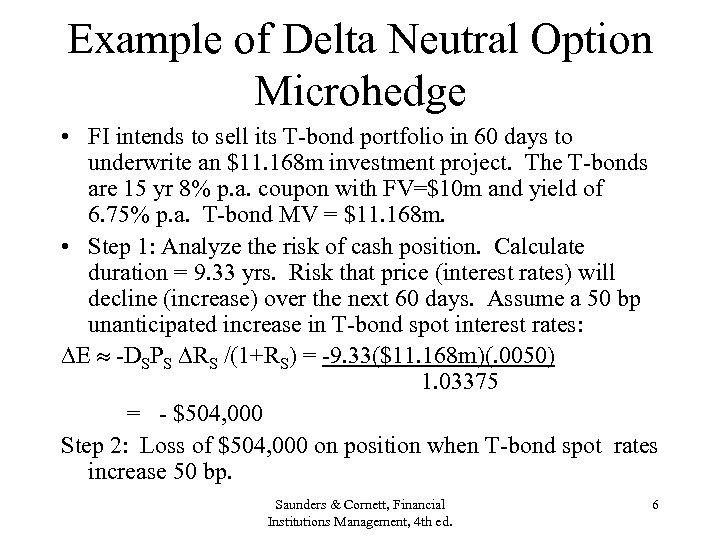Example of Delta Neutral Option Microhedge • FI intends to sell its T-bond portfolio in 60 days to underwrite an \$11. 168 m investment project. The T-bonds are 15 yr 8% p. a. coupon with FV=\$10 m and yield of 6. 75% p. a. T-bond MV = \$11. 168 m. • Step 1: Analyze the risk of cash position. Calculate duration = 9. 33 yrs. Risk that price (interest rates) will decline (increase) over the next 60 days. Assume a 50 bp unanticipated increase in T-bond spot interest rates: E -DSPS RS /(1+RS) = -9. 33(\$11. 168 m)(. 0050) 1. 03375 = - \$504, 000 Step 2: Loss of \$504, 000 on position when T-bond spot rates increase 50 bp. Saunders & Cornett, Financial Institutions Management, 4 th ed. 6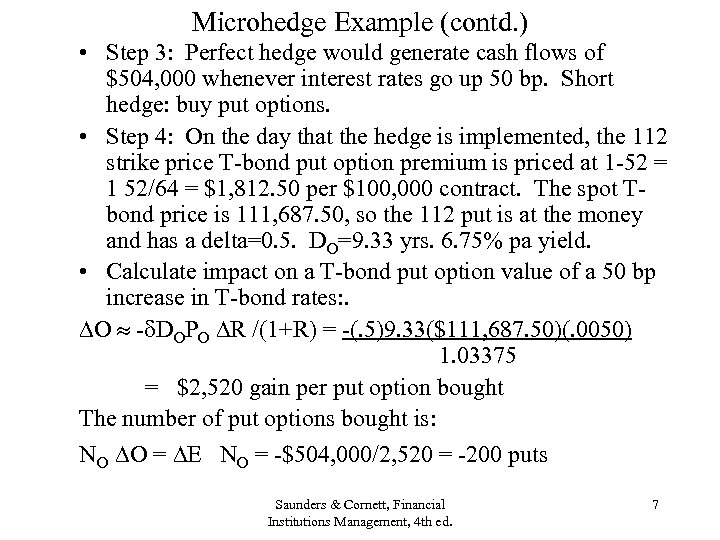Microhedge Example (contd. ) • Step 3: Perfect hedge would generate cash flows of \$504, 000 whenever interest rates go up 50 bp. Short hedge: buy put options. • Step 4: On the day that the hedge is implemented, the 112 strike price T-bond put option premium is priced at 1 -52 = 1 52/64 = \$1, 812. 50 per \$100, 000 contract. The spot Tbond price is 111, 687. 50, so the 112 put is at the money and has a delta=0. 5. DO=9. 33 yrs. 6. 75% pa yield. • Calculate impact on a T-bond put option value of a 50 bp increase in T-bond rates: . O - DOPO R /(1+R) = -(. 5)9. 33(\$111, 687. 50)(. 0050) 1. 03375 = \$2, 520 gain per put option bought The number of put options bought is: NO O = E NO = -\$504, 000/2, 520 = -200 puts Saunders & Cornett, Financial Institutions Management, 4 th ed. 7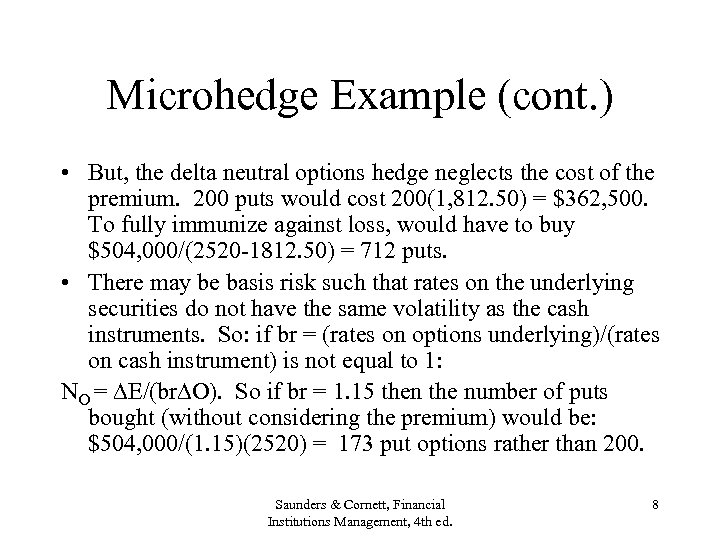Microhedge Example (cont. ) • But, the delta neutral options hedge neglects the cost of the premium. 200 puts would cost 200(1, 812. 50) = \$362, 500. To fully immunize against loss, would have to buy \$504, 000/(2520 -1812. 50) = 712 puts. • There may be basis risk such that rates on the underlying securities do not have the same volatility as the cash instruments. So: if br = (rates on options underlying)/(rates on cash instrument) is not equal to 1: NO = E/(br O). So if br = 1. 15 then the number of puts bought (without considering the premium) would be: \$504, 000/(1. 15)(2520) = 173 put options rather than 200. Saunders & Cornett, Financial Institutions Management, 4 th ed. 8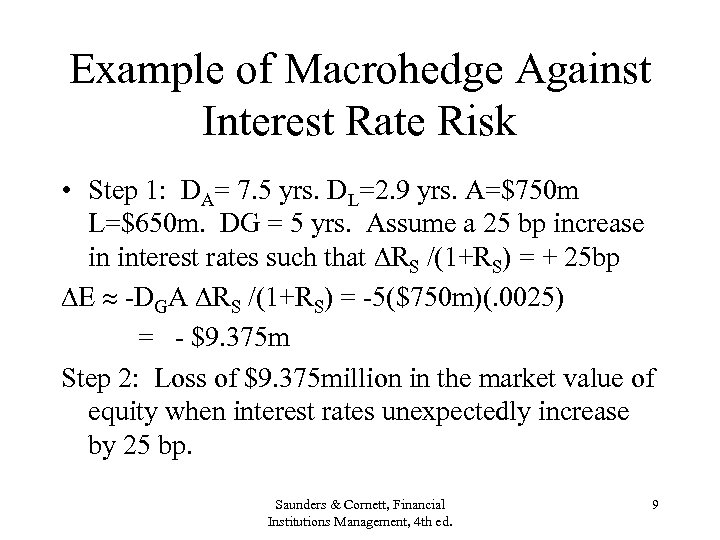Example of Macrohedge Against Interest Rate Risk • Step 1: DA= 7. 5 yrs. DL=2. 9 yrs. A=\$750 m L=\$650 m. DG = 5 yrs. Assume a 25 bp increase in interest rates such that RS /(1+RS) = + 25 bp E -DGA RS /(1+RS) = -5(\$750 m)(. 0025) = - \$9. 375 m Step 2: Loss of \$9. 375 million in the market value of equity when interest rates unexpectedly increase by 25 bp. Saunders & Cornett, Financial Institutions Management, 4 th ed. 9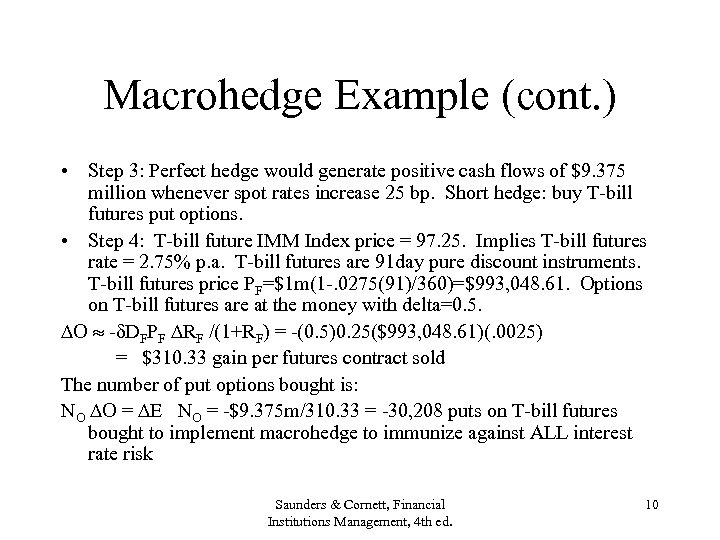Macrohedge Example (cont. ) • Step 3: Perfect hedge would generate positive cash flows of \$9. 375 million whenever spot rates increase 25 bp. Short hedge: buy T-bill futures put options. • Step 4: T-bill future IMM Index price = 97. 25. Implies T-bill futures rate = 2. 75% p. a. T-bill futures are 91 day pure discount instruments. T-bill futures price PF=\$1 m(1 -. 0275(91)/360)=\$993, 048. 61. Options on T-bill futures are at the money with delta=0. 5. O - DFPF RF /(1+RF) = -(0. 5)0. 25(\$993, 048. 61)(. 0025) = \$310. 33 gain per futures contract sold The number of put options bought is: NO O = E NO = -\$9. 375 m/310. 33 = -30, 208 puts on T-bill futures bought to implement macrohedge to immunize against ALL interest rate risk Saunders & Cornett, Financial Institutions Management, 4 th ed. 10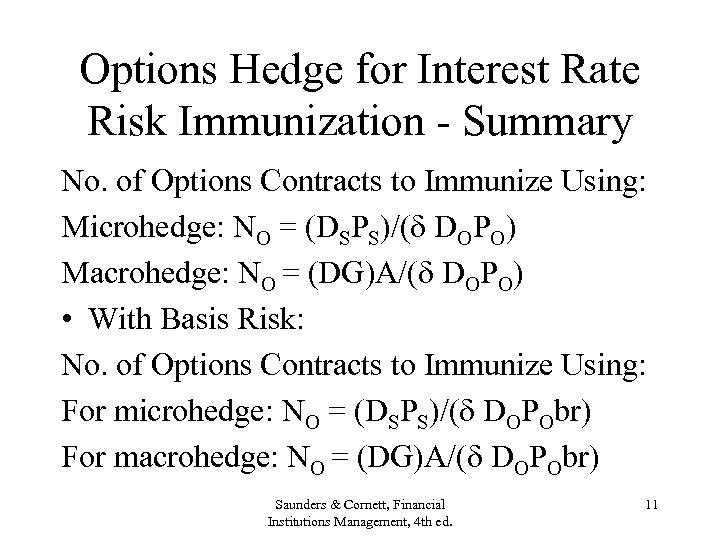Options Hedge for Interest Rate Risk Immunization - Summary No. of Options Contracts to Immunize Using: Microhedge: NO = (DSPS)/( DOPO) Macrohedge: NO = (DG)A/( DOPO) • With Basis Risk: No. of Options Contracts to Immunize Using: For microhedge: NO = (DSPS)/( DOPObr) For macrohedge: NO = (DG)A/( DOPObr) Saunders & Cornett, Financial Institutions Management, 4 th ed. 11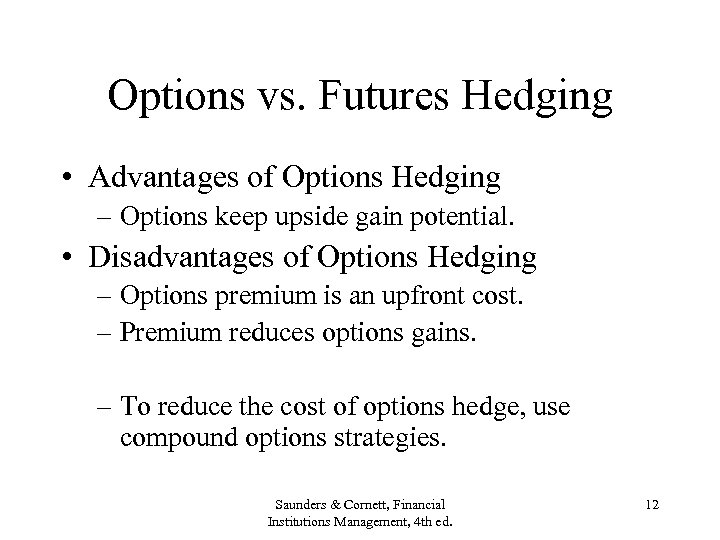Options vs. Futures Hedging • Advantages of Options Hedging – Options keep upside gain potential. • Disadvantages of Options Hedging – Options premium is an upfront cost. – Premium reduces options gains. – To reduce the cost of options hedge, use compound options strategies. Saunders & Cornett, Financial Institutions Management, 4 th ed. 12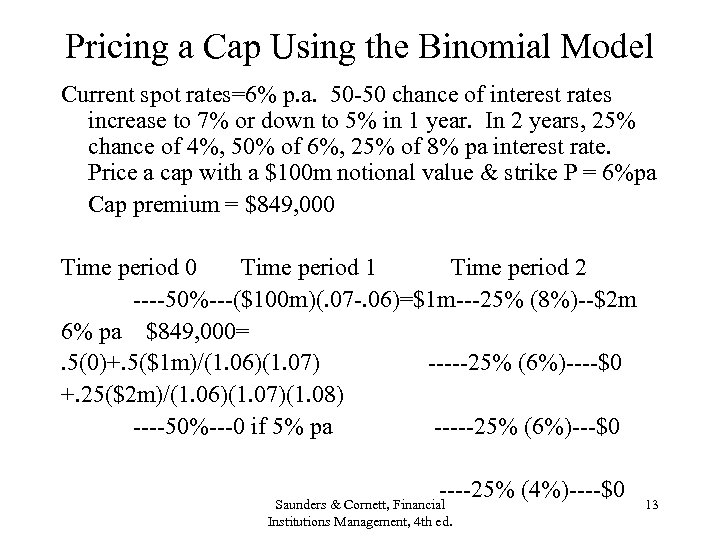Pricing a Cap Using the Binomial Model Current spot rates=6% p. a. 50 -50 chance of interest rates increase to 7% or down to 5% in 1 year. In 2 years, 25% chance of 4%, 50% of 6%, 25% of 8% pa interest rate. Price a cap with a \$100 m notional value & strike P = 6%pa Cap premium = \$849, 000 Time period 1 Time period 2 ----50%---(\$100 m)(. 07 -. 06)=\$1 m---25% (8%)--\$2 m 6% pa \$849, 000=. 5(0)+. 5(\$1 m)/(1. 06)(1. 07) -----25% (6%)----\$0 +. 25(\$2 m)/(1. 06)(1. 07)(1. 08) ----50%---0 if 5% pa -----25% (6%)---\$0 ----25% (4%)----\$0 Saunders & Cornett, Financial Institutions Management, 4 th ed. 13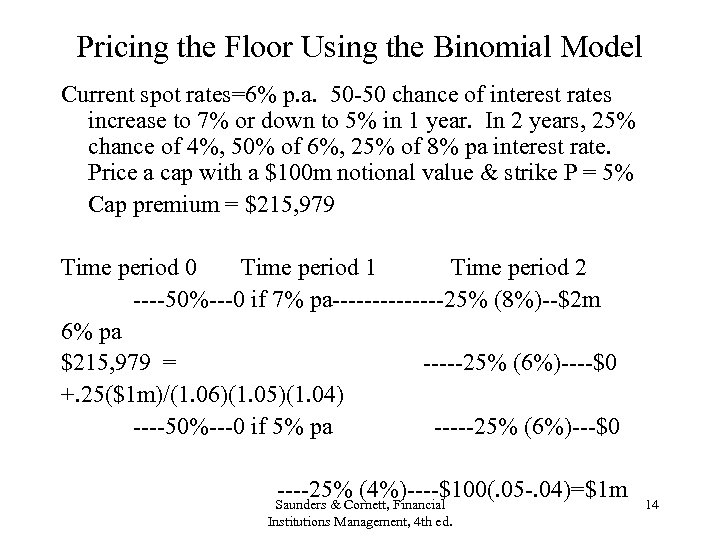Pricing the Floor Using the Binomial Model Current spot rates=6% p. a. 50 -50 chance of interest rates increase to 7% or down to 5% in 1 year. In 2 years, 25% chance of 4%, 50% of 6%, 25% of 8% pa interest rate. Price a cap with a \$100 m notional value & strike P = 5% Cap premium = \$215, 979 Time period 0 Time period 1 Time period 2 ----50%---0 if 7% pa-------25% (8%)--\$2 m 6% pa \$215, 979 = -----25% (6%)----\$0 +. 25(\$1 m)/(1. 06)(1. 05)(1. 04) ----50%---0 if 5% pa -----25% (6%)---\$0 ----25% (4%)----\$100(. 05 -. 04)=\$1 m Saunders & Cornett, Financial Institutions Management, 4 th ed. 14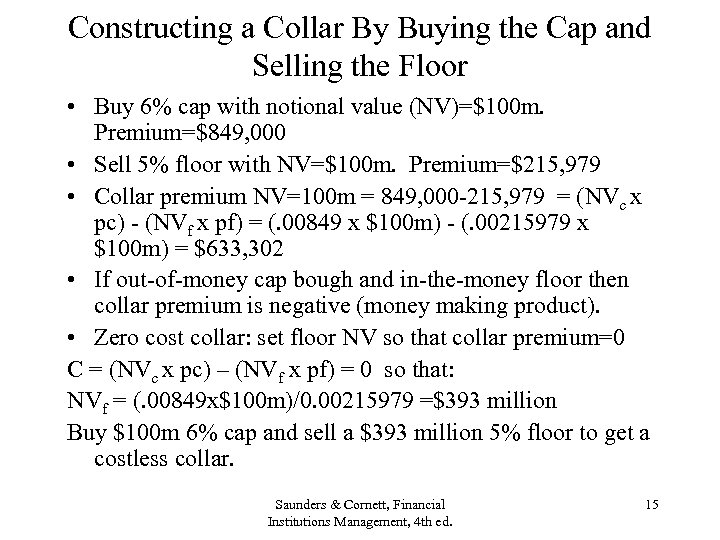Constructing a Collar By Buying the Cap and Selling the Floor • Buy 6% cap with notional value (NV)=\$100 m. Premium=\$849, 000 • Sell 5% floor with NV=\$100 m. Premium=\$215, 979 • Collar premium NV=100 m = 849, 000 -215, 979 = (NVc x pc) - (NVf x pf) = (. 00849 x \$100 m) - (. 00215979 x \$100 m) = \$633, 302 • If out-of-money cap bough and in-the-money floor then collar premium is negative (money making product). • Zero cost collar: set floor NV so that collar premium=0 C = (NVc x pc) – (NVf x pf) = 0 so that: NVf = (. 00849 x\$100 m)/0. 00215979 =\$393 million Buy \$100 m 6% cap and sell a \$393 million 5% floor to get a costless collar. Saunders & Cornett, Financial Institutions Management, 4 th ed. 15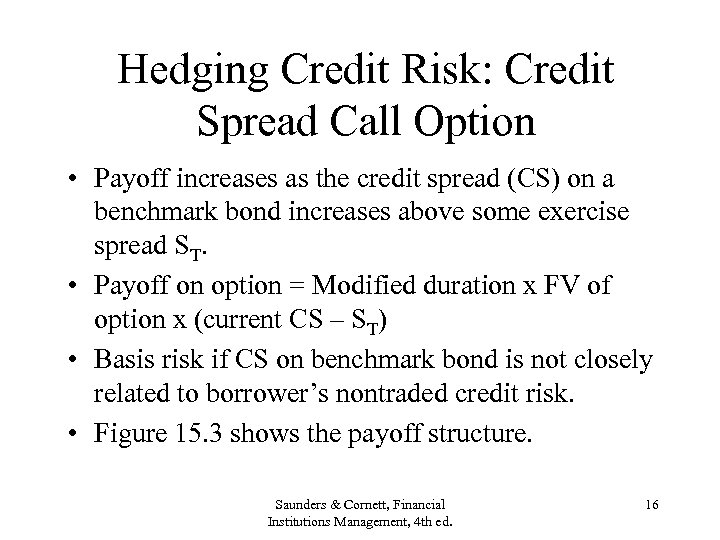Hedging Credit Risk: Credit Spread Call Option • Payoff increases as the credit spread (CS) on a benchmark bond increases above some exercise spread ST. • Payoff on option = Modified duration x FV of option x (current CS – ST) • Basis risk if CS on benchmark bond is not closely related to borrower’s nontraded credit risk. • Figure 15. 3 shows the payoff structure. Saunders & Cornett, Financial Institutions Management, 4 th ed. 16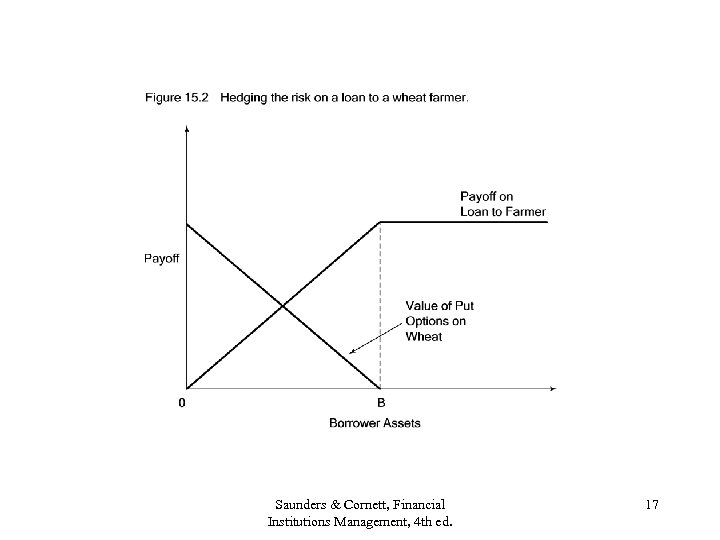Saunders & Cornett, Financial Institutions Management, 4 th ed. 17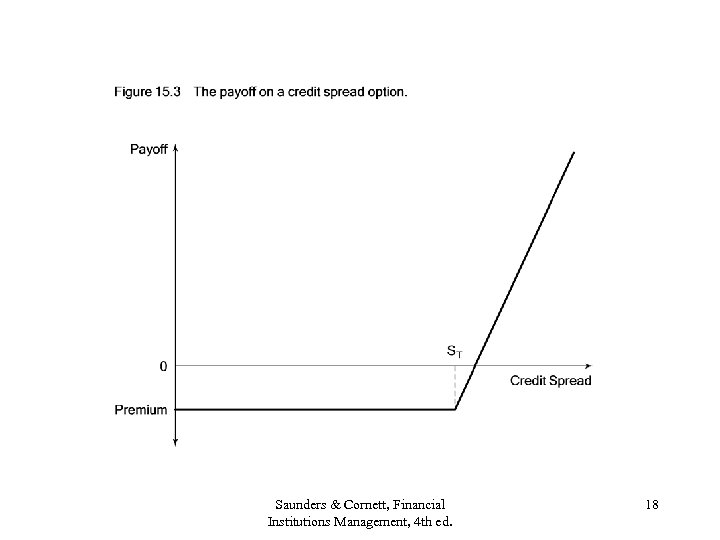Saunders & Cornett, Financial Institutions Management, 4 th ed. 18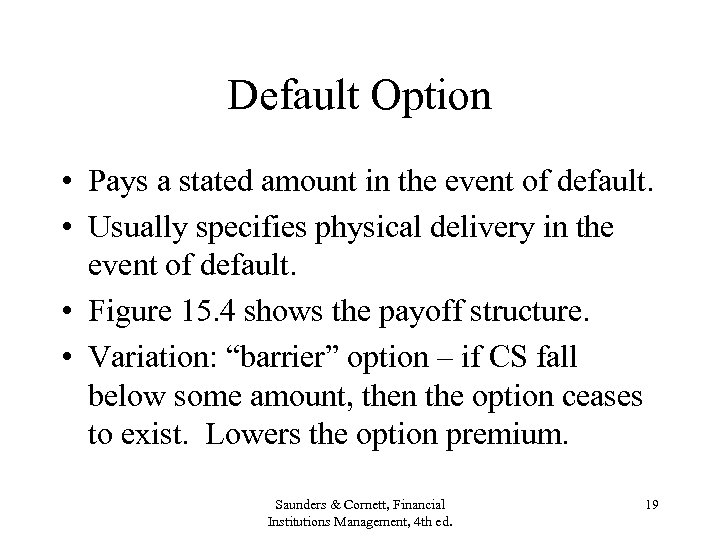Default Option • Pays a stated amount in the event of default. • Usually specifies physical delivery in the event of default. • Figure 15. 4 shows the payoff structure. • Variation: “barrier” option – if CS fall below some amount, then the option ceases to exist. Lowers the option premium. Saunders & Cornett, Financial Institutions Management, 4 th ed. 19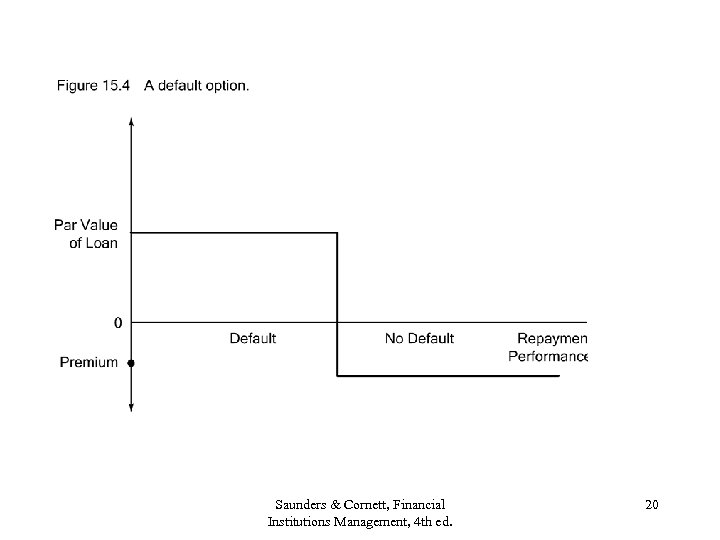Saunders & Cornett, Financial Institutions Management, 4 th ed. 20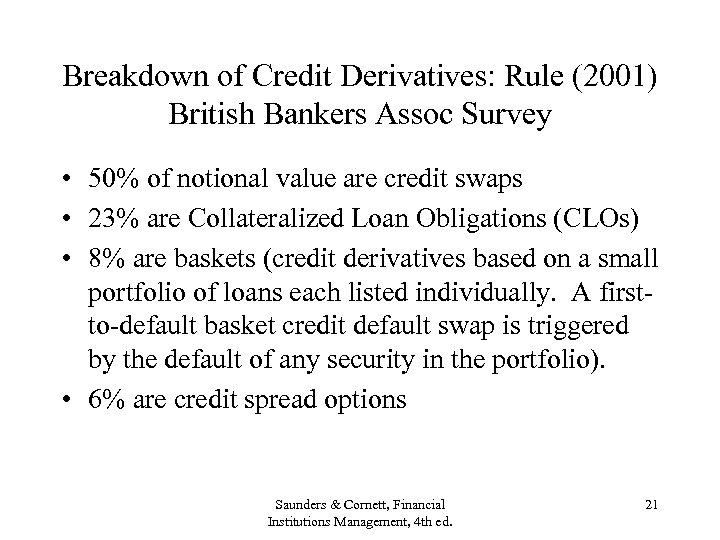Breakdown of Credit Derivatives: Rule (2001) British Bankers Assoc Survey • 50% of notional value are credit swaps • 23% are Collateralized Loan Obligations (CLOs) • 8% are baskets (credit derivatives based on a small portfolio of loans each listed individually. A firstto-default basket credit default swap is triggered by the default of any security in the portfolio). • 6% are credit spread options Saunders & Cornett, Financial Institutions Management, 4 th ed. 21